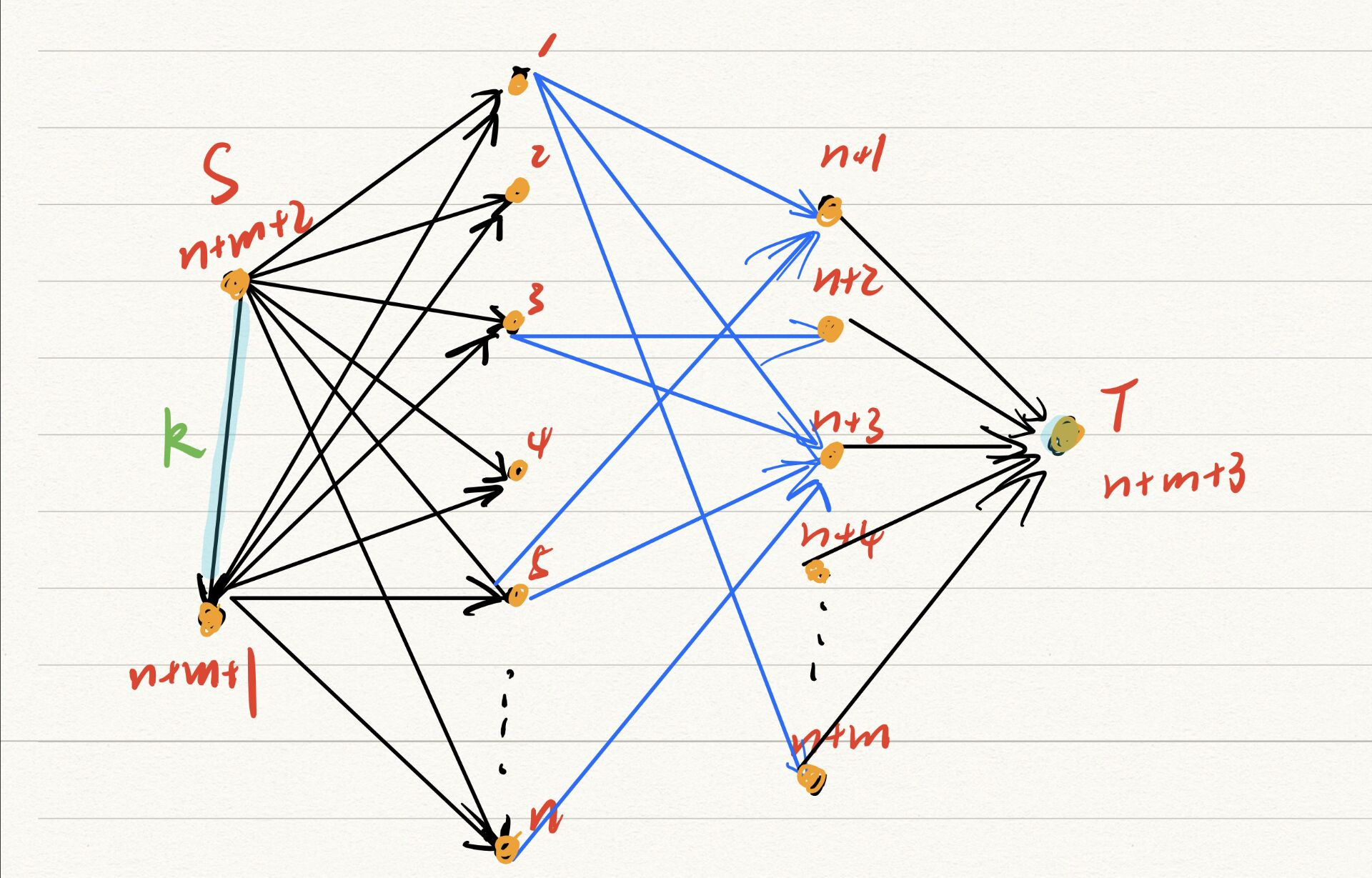2U Nov 21, 2019
•# Magic Potion

There are n heroes and mm monsters living in an island. The monsters became very vicious these days, so the heroes decided to diminish the monsters in the island. However, the i-th hero can only kill one monster belonging to the set Mi​. Joe, the strategist, has k bottles of magic potion, each of which can buff one hero’s power and let him be able to kill one more monster. Since the potion is very powerful, a hero can only take at most one bottle of potion.

Please help Joe find out the maximum number of monsters that can be killed by the heroes if he uses the optimal strategy.

### Input

The first line contains three integers n,m,k (1≤n,m,k≤500) —— the number of heroes, the number of monsters and the number of bottles of potion.

Each of the next n lines contains one integer ti​, the size of Mi​, and the following ti​ integers Mi,j​ (1≤j≤ti​), the indices (1-based) of monsters that can be killed by the i-th hero (1≤ti​≤m,1≤Mi,j​≤m).

### Output

Print the maximum number of monsters that can be killed by the heroes.

### 样例输入1

3 5 2
4 1 2 3 5
2 2 5
2 1 2

### 样例输出1

4

### 样例输入2

5 10 2
2 3 10
5 1 3 4 6 10
5 3 4 6 8 9
3 1 9 10
5 1 3 6 7 10

### 样例输出2

7

### 题目来源

ACM-ICPC Nanjing Onsite 2018

### 题解#include <bits/stdc++.h>
using namespace std;

typedef long long ll;
const int maxn = 1e6 + 10;
const int maxm = 1e6 + 10;
const int inf = 0x3f3f3f3f;
struct edge { int to, flow, next; } e[maxm];

{
int x = 0, f = 1; char ch = getchar();
while (ch < '0' || ch > '9') { if (ch == '-') f = -1; ch = getchar(); }
while (ch >= '0' && ch <= '9') { x = (x << 3) + (x << 1) + ch - '0'; ch = getchar(); }
return x * f;
}

void addEdge(int u, int v, int w)
{
e[cnt].to = v;
e[cnt].flow = w;
}

bool bfs(int s, int t)
{
queue<int> q;
memset(dep, -1, sizeof(dep));
dep[s] = 0;
q.push(s);
while (!q.empty())
{
int u = q.front();
q.pop();
for (int i = head[u]; ~i; i = e[i].next)
{
int v = e[i].to;
if (dep[v] == -1 && e[i].flow)
{
q.push(v);
dep[v] = dep[u] + 1;
}
}
}
return ~dep[t];
}

int dfs(int u, int t, int rflow)
{
if (u == t) return rflow;
int res = 0;
for (int i = cur[u]; ~i && rflow; i = e[i].next)
{
int v = e[i].to;
if (dep[v] != dep[u] + 1 || !e[i].flow) continue;
cur[u] = i;
int flow = dfs(v, t, min(e[i].flow, rflow));
e[i].flow -= flow;
e[i ^ 1].flow += flow;
rflow -= flow;
res += flow;
}
if (!res) dep[u] = -1;
return res;
}

int dinic(int s, int t)
{
int res = 0;
while (bfs(s, t))
{
res += dfs(s, t, inf);
}
return res;
}

int main()
{
addEdge(n + m + 2, n + m + 1, k);
addEdge(n + m + 1, n + m + 2, 0);
for (int i = 1; i <= n; i++)
{
addEdge(n + m + 2, i, 1);
addEdge(i, n + m + 2, 0);
addEdge(n + m + 1, i, 1);
addEdge(i, n + m + 1, 0);
while (t--)
{
}